Definitions of Square Dance Calls and Concepts

Index -->  Plus  |  A1  |  A2  |  C1  |  C2  |  C3A  |  C3B  |  C4  |  NOL  |
Definitions (Text Only) -->  Plus  |  A1  |  A2  |  C1  |  C2  |  C3A  |  C3B  |  C4  |  NOL  |
 Find call:

Rotate [C1,C2,C3B]
 C1: C2: C3B:Rotates (Rotate, Reverse Rotate, Single Rotate, and Reverse Single Rotate) are listed on different challenge lists according to the starting formation. EN: 10
Rotates (Rotate, Reverse Rotate, Single Rotate, Reverse Single Rotate) \$B\$O(B, \$B%3!<%k\$r;O\$a\$k(B formation \$B\$K=>\$C\$F(B, \$B0[\$J\$k(B challenge list \$B\$K\$"\$j\$^\$9(B. JP: 10

At C1, EN: 20
C1 \$B\$G\$O(B, JP: 20
Rotates can only be called from a Generalized Squared Set, or from similar formations (e.g., from Columns, the Ends only). EN: 30
Rotates \$B\$O(B, \$B0lHLE*\$J(B squared set, \$B\$^\$?\$O(B, \$B;w\$?(B formation (\$BNc\$(\$P(B, Column \$B\$N(B Ends \$B\$@\$1(B) \$B\$+\$i\$N\$_%3!<%k\$5\$l\$^\$9(B. JP: 30
At C2, EN: 40
C2 \$B\$G\$O(B, JP: 40
Rotates can also be called from Parallel Lines or from a 2 x 2 (e.g., In Your Block Single Rotate 1/4). EN: 50
Rotates \$B\$O(B, \$BJ?9T\$J(B Lines \$B\$^\$?\$O(B 2 x 2 (\$BNc\$(\$P(B, In Your Block Single Rotate 1/4) \$B\$+\$i\$b%3!<%k\$5\$l\$^\$9(B. JP: 50
At C3B, EN: 60
C3B \$B\$G\$O(B, JP: 60
Rotates can also be called from Columns. EN: 70
Rotates \$B\$O(B, Columns \$B\$+\$i\$b%3!<%k\$5\$l\$^\$9(B. JP: 70

Procedure for dancing Rotates: EN: 80
Rotates \$B\$rMY\$k\$?\$a\$NJ}K!(B: JP: 80
1. Identify the center point of your setup. EN: 90
\$B<+J,\$N(B setup \$B\$NCf?4E@\$r3NG'\$7\$^\$9(B. JP: 90

The center point of your setup is the very center point of the square (commonly called the "flagpole center") except in the following cases: EN: 100
\$B<+J,\$N(B setup \$B\$NCf?4E@\$H\$O(B, \$B0J2<\$N>l9g\$r=|\$-(B, square \$B\$N??\$sCf(B (\$B\$h\$/(B "flagpole center (flagpole: \$B4z\$6\$*(B)" \$B\$H8@\$o\$l\$^\$9(B) \$B\$G\$9(B: JP: 100

1. If you are in a 2 x 4 formation and are in Lines, the center point is the Center of each Box (i.e., work Split). If you are in a 2 x 4 T-Bone, the center point for the Column dancers is the flagpole center, whereas the Line dancers are working Split. EN: 110
\$B\$b\$7(B, 2 x 4 formation \$B\$K\$\$\$F(B Lines \$B\$G\$"\$l\$P(B, \$BCf?4E@\$O(B each Box \$B\$N(B Center (\$B\$9\$J\$o\$A(B, Split \$B\$GF0\$-\$^\$9(B) \$B\$G\$9(B. \$B\$b\$7(B, 2 x 4 \$B\$N(B T-Bone \$B\$J\$i(B, Line \$B\$N?M\$,(B Split \$B\$GF0\$/\$N\$KBP\$7\$F(B, Column \$B\$N?M\$NCf?4E@\$O(B flagpole center (squared set \$B\$NCf?4(B) \$B\$G\$9(B.  JP: 110
2. If Rotate is preceded by the term Split, then divide your formation into two equal subformations. The center point is the Center of your subformation. From a 2 x 4, Split means to divide the setup into two 2 x 2s, not two 1 x 4s. EN: 120
\$B\$b\$7(B, Rotate \$B\$NA0\$K(B Split \$B\$N8@MU\$,\$"\$l\$P(B, formation \$B\$r(B 2 \$B\$D\$NEy\$7\$\$(B subformation \$B\$KJ,\$1\$^\$9(B. \$BCf?4E@\$O(B subformation \$B\$N(B Center \$B\$G\$9(B. 2 x 4 \$B\$+\$i(B, Split \$B\$O(B setup \$B\$r(B 2 \$B\$D\$N(B 2 x 2 \$B\$XJ,\$1\$k\$3\$H\$r0UL#\$7(B, 2 \$B\$D\$N(B 1 x 4 \$B\$G\$O\$"\$j\$^\$;\$s(B. JP: 120
3. If Rotate is preceded by the name of a formation in which to work, the center point is the Center of that formation. For example, from Columns consider 'Each Column Single Rotate 1/4'. In this case, the center point is the Center of the 1 x 4 setup. EN: 130
\$B\$b\$7(B, Rotate \$B\$NA0\$K(B, \$BF0\$-\$r9T\$&(B formation \$B\$NL>A0\$,\$"\$l\$P(B, \$BCf?4E@\$O\$=\$N(B formation \$B\$N(B Center \$B\$G\$9(B. \$BNc\$(\$P(B, Columns \$B\$+\$i\$N(B 'Each Column Single Rotate 1/4' \$B\$r9M\$(\$F\$_\$^\$7\$g\$&(B. \$B\$3\$N>l9g(B, \$BCf?4E@\$O(B 1 x 4 setup \$B\$N(B Center \$B\$G\$9(B. JP: 130
2. Turn 1/4 relative to that center point according to the following rules: EN: 140
\$B2<5-\$N%k!<%k\$K=>\$C\$F(B, \$B3NG'\$7\$?Cf?4E@\$NJ}\$X(B 1/4 \$B8~\$-\$rJQ\$(\$^\$9(B: JP: 140

 Rotate As Couples 1/4 To Promenade Reverse Rotate As Couples 1/4 To Reverse Promenade Single Rotate Individually 1/4 To Promenade EN: 150 Individually 1/4 To Promenade JP: 150 Reverse Single Rotate Individually 1/4 To Reverse Promenade EN: 160 Individually 1/4 To Reverse Promenade JP: 160

Turn 1/4 so that your left shoulder is toward the Center of your formation. EN: 170
\$B:8(B\$B8*\$,<+J,\$N(B formation \$B\$N(B Center \$B\$X8~\$/\$h\$&\$K(B 1/4 \$B\$=\$N>l\$G2s\$j\$^\$9(B. JP: 170
Turn 1/4 so that your right shoulder is toward the Center of your formation. EN: 180
\$B1&(B\$B8*\$,<+J,\$N(B formation \$B\$N(B Center \$B\$X8~\$/\$h\$&\$K(B 1/4 \$B\$=\$N>l\$G2s\$j\$^\$9(B. JP: 180
3. Counter Rotate the given fraction around that center point. EN: 190
\$BCf?4E@\$N2s\$j\$G(B Counter Rotate \$B\$rM?\$(\$i\$l\$?(B fraction \$B\$@\$19T\$\$\$^\$9(B. JP: 190

From a Squared Set, active dancers work around the outside of the set. The inactive dancers should move into the center of the set. EN: 200
Squared Set \$B\$+\$i(B, \$B%"%/%F%#%V\$N%@%s%5!<\$O(B set \$B\$N30B&\$r2s\$k\$h\$&\$KF0\$-\$^\$9(B. \$B%"%/%F%#%V\$G\$J\$\$?M\$O(B set \$B\$N(B Center \$B\$XF0\$+\$M\$P\$J\$j\$^\$;\$s(B.  JP: 200

When dancing Rotates, there are 3 things to listen for: EN: 210
Rotates \$B\$rMY\$k\$H\$-\$K\$O(B, \$B JP: 210

1. Single or not; EN: 220
Single \$B\$,\$"\$k\$+\$J\$\$\$+(B; JP: 220
2. Reverse or not; EN: 230
Reverse \$B\$,\$"\$k\$+\$J\$\$\$+(B; JP: 230
3. How far. EN: 240
\$B\$I\$l\$@\$1F0\$/\$+(B. JP: 240

C1 Rotates (

from Squared Set EN: 250
Squared Set \$B\$+\$i(B JP: 250
)

Rotate fraction [C1]:

(Those designated) As Couples 1/4 To Promenade & Counter Rotate the given fraction around the outside of the set as non-designated dancers (if any) move into the Center. EN: 260
(\$B;X<(\$5\$l\$??M\$,(B) \$B%+%C%W%k\$G(B, promenade \$B\$NJ}\$X(B 1/4 \$B8~\$-\$rJQ\$((B; Counter Rotate \$B\$rM?\$(\$i\$l\$?(B fraction \$B\$@\$1(B set \$B\$N30\$r2s\$k\$h\$&\$K9T\$\$(B, \$B;X<(\$5\$l\$J\$+\$C\$??M\$O(B (\$B\$b\$7(B, \$B\$\$\$l\$P(B) Center \$B\$XF0\$-\$^\$9(B. JP: 260

Reverse Rotate fraction [C1]:

(Those designated) As Couples 1/4 To Reverse Promenade & Counter Rotate the given fraction around the outside of the set as non-designated dancers (if any) move into the Center. EN: 270
(\$B;X<(\$5\$l\$??M\$,(B) \$B%+%C%W%k\$G(B, \$B5U(B promenade \$B\$NJ}\$X(B 1/4 \$B8~\$-\$rJQ\$((B; Counter Rotate \$B\$rM?\$(\$i\$l\$?(B fraction \$B\$@\$1(B set \$B\$N30\$r2s\$k\$h\$&\$K9T\$\$(B, \$B;X<(\$5\$l\$J\$+\$C\$??M\$O(B (\$B\$b\$7(B, \$B\$\$\$l\$P(B) Center \$B\$XF0\$-\$^\$9(B. JP: 270

Single Rotate fraction [C1]:

(Those designated) Individually 1/4 To Promenade & Counter Rotate the given fraction around the outside of the set as non-designated dancers (if any) move into the Center. EN: 280
(\$B;X<(\$5\$l\$??M\$,(B) 1 \$B?M\$G(B, promenade \$B\$NJ}\$X(B 1/4 \$B8~\$-\$rJQ\$((B; Counter Rotate \$B\$rM?\$(\$i\$l\$?(B fraction \$B\$@\$1(B set \$B\$N30\$r2s\$k\$h\$&\$K9T\$\$(B, \$B;X<(\$5\$l\$J\$+\$C\$??M\$O(B (\$B\$b\$7(B, \$B\$\$\$l\$P(B) Center \$B\$XF0\$-\$^\$9(B. JP: 280

Reverse Single Rotate fraction [C1]:

(Those designated) Individually 1/4 To Reverse Promenade & Counter Rotate the given fraction around the outside of the set as non-designated dancers (if any) move into the Center. EN: 290
(\$B;X<(\$5\$l\$??M\$,(B) 1 \$B?M\$G(B, \$B5U(B promenade \$B\$NJ}\$X(B 1/4 \$B8~\$-\$rJQ\$((B; Counter Rotate \$B\$rM?\$(\$i\$l\$?(B fraction \$B\$@\$1(B set \$B\$N30\$r2s\$k\$h\$&\$K9T\$\$(B, \$B;X<(\$5\$l\$J\$+\$C\$??M\$O(B (\$B\$b\$7(B, \$B\$\$\$l\$P(B) Center \$B\$XF0\$-\$^\$9(B. JP: 290

\$BCm
• Do not Face In or Bend The Line after a Rotate unless the caller explicitly tells you to do so. EN: 300
Rotate \$B\$N8e(B, \$B%3!<%i!<\$N;X<(\$,\$J\$\$8B\$j(B, Face In \$B\$d(B Bend the Line \$B\$r\$7\$J\$\$\$G\$/\$@\$5\$\$(B.  JP: 300
• Non-designated dancers move into the center of the set without changing their facing direction. EN: 310
\$B;X<(\$5\$l\$J\$+\$C\$??M\$O(B, set \$B\$N(B center \$B\$X(B, \$B8~\$-\$rJQ\$(\$:\$KF0\$-\$^\$9(B.  JP: 310
• Rotate 1/4 (
from Squared Set EN: 320
Squared Set \$B\$+\$i(B JP: 320
)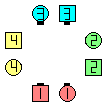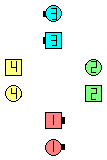Heads Rotate 1/4\$B\$NA0(B As Couples 1/4 To Promenade\$B\$N8e(B Counter Rotate 1/4\$B\$N8e(B (\$B=*\$o\$j(B)
Single Rotate 1/2 (
from Squared Set EN: 320
Squared Set \$B\$+\$i(B JP: 320
)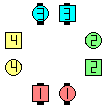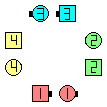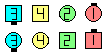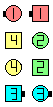Heads Single Rotate 1/2\$B\$NA0(B 1/4 To Promenade\$B\$N8e(B Counter Rotate 1/4\$B\$N8e(B Counter Rotate 1/4 (again)\$B\$N8e(B (\$B=*\$o\$j(B)

C2 Rotates (

from Parallel Lines, or from a 2 x 2 but not from Columns EN: 340
\$BJ?9T\$J(B Line \$B\$+\$i(B, \$B\$^\$?\$O(B 2 x 2 (\$B\$?\$@\$7(B Column \$B\$G\$O\$J\$/(B) \$B\$+\$i(B): JP: 340
):

Work in each Box of 4. EN: 350
4 \$B?M\$N(B each Box \$B\$GF0\$-\$^\$9(B. JP: 350

Rotate fraction[C2]:

As Couples 1/4 To Promenade and (working in the resulting Line of 4) Counter Rotate the given fraction. This is the same as As Couples Zag-Zig; Lockit n times (where n is the number of quarters within the given fraction: n=1 for 1/4, n=2 for 1/2, n=3 for 3/4, etc.). EN: 360
\$B%+%C%W%k\$G(B, promenade \$B\$NJ}\$X(B 1/4 \$B8~\$-\$rJQ\$((B, (\$B7k2L\$H\$7\$F\$G\$-\$?(B 4 \$B?M\$N(B Line \$B\$GF0\$/\$h\$&\$K(B) Counter Rotate \$B\$rM?\$(\$i\$l\$?(B fraction \$B\$@\$19T\$\$\$^\$9(B. \$B\$3\$l\$O(B, As Couples Zag-Zig \$B\$r\$7\$F(B; Lockit \$B\$r(B n \$B2s\$9\$k\$3\$H\$HF1\$8\$G\$9(B (\$B\$3\$3\$G(B n \$B\$O(B, \$BM?\$(\$i\$l\$?(B fraction \$B\$N(B 1/4 \$B\$N?t(B: 1/4 \$B\$G\$O(B n=1, 1/2 \$B\$G\$O(B n=2, 3/4 \$B\$G\$O(B n=3, etc \$B\$G\$9(B). JP: 360

Reverse Rotate fraction [C2]:

As Couples 1/4 To Reverse Promenade and (working in the resulting Line of 4) Counter Rotate the given fraction. Same as As Couples Zig-Zag; Lockit n times (where n is the number of quarters within the given fraction). EN: 370
\$B%+%C%W%k\$G(B, \$B5U(B promenade \$B\$NJ}\$X(B 1/4 \$B8~\$-\$rJQ\$((B, (\$B7k2L\$H\$7\$F\$G\$-\$?(B 4 \$B?M\$N(B Line \$B\$GF0\$/\$h\$&\$K(B) Counter Rotate \$B\$rM?\$(\$i\$l\$?(B fraction \$B\$@\$19T\$\$\$^\$9(B. \$B\$3\$l\$O(B, As Couples Zig-Zag \$B\$r\$7\$F(B; Lockit \$B\$r(B n \$B2s\$9\$k\$3\$H\$HF1\$8\$G\$9(B (\$B\$3\$3\$G(B n \$B\$O(B, \$BM?\$(\$i\$l\$?(B fraction \$B\$N(B 1/4 \$B\$N?t\$G\$9(B). JP: 370

Single Rotate fraction [C2]:

Individually 1/4 To Promenade and Box Counter Rotate the given fraction. Same as Zag-Zig; Box|Split Counter Rotate the given fractionEN: 380
1 \$B?M\$G(B, promenade \$B\$NJ}\$X(B 1/4 \$B8~\$-\$rJQ\$((B, Box Counter Rotate \$B\$rM?\$(\$i\$l\$?(B fraction \$B\$@\$19T\$\$\$^\$9(B. \$B\$3\$l\$O(B, Zag-Zig \$B\$r\$7\$F(B; Box|Split Counter Rotate \$B\$rM?\$(\$i\$l\$?(B fraction \$B\$@\$19T\$&\$3\$H\$HF1\$8\$G\$9(B. JP: 380

Reverse Single Rotate fraction[C2]:

Individually 1/4 To Reverse Promenade and Box Counter Rotate the given fraction. Same as Zig-Zag; Box|Split Counter Rotate the given fractionEN: 390
1 \$B?M\$G(B, \$B5U(B promenade \$B\$NJ}\$X(B 1/4 \$B8~\$-\$rJQ\$((B, Box Counter Rotate \$B\$rM?\$(\$i\$l\$?(B fraction \$B\$@\$19T\$\$\$^\$9(B. \$B\$3\$l\$O(B, Zig-Zag \$B\$r\$7\$F(B; Box|Split Counter Rotate \$B\$rM?\$(\$i\$l\$?(B fraction \$B\$@\$19T\$&\$3\$H\$HF1\$8\$G\$9(B. JP: 390

Reverse Rotate 1/4 (
from Parallel Lines EN: 400
\$BJ?9T\$J(B Line \$B\$+\$i(B JP: 400
):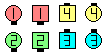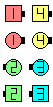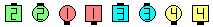Reverse Rotate 1/4\$B\$NA0(B As Couples 1/4 To Reverse Promenade\$B\$N8e(B Line of 4 Counter Rotate 1/4\$B\$N8e(B (\$B=*\$o\$j(B)
Reverse Single Rotate 1/2 (
from Parallel Lines EN: 400
\$BJ?9T\$J(B Line \$B\$+\$i(B JP: 400
):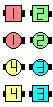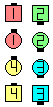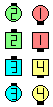Reverse Single Rotate 1/2\$B\$NA0(B 1/4 To Reverse Promenade\$B\$N8e(B Split Counter Rotate 1/2\$B\$N8e(B (\$B=*\$o\$j(B)

Cheat for Single Rotate 1/4 (from Lines): EN: 420
Single Rotate 1/4 (Line \$B\$+\$i(B) \$B\$N(B Cheat: JP: 420
Leaders Left-faced U-Turn Back; Belles Walk; Beaus Dodge. EN: 430
Leaders \$B\$O(B Left-faced U-Turn Back \$B\$r\$7\$F(B; Belles Walk; Beaus Dodge. JP: 430
Cheat for Reverse Single Rotate 1/4 (from Lines): EN: 440
Reverse Single Rotate 1/4 (Line \$B\$+\$i(B) \$B\$N(B Cheat: JP: 440
Leaders Right-faced U-Turn Back; Beaus Walk; Belles Dodge. EN: 450
Leaders \$B\$O(B Right-faced U-Turn Back \$B\$r\$7\$F(B; Beaus Walk; Belles Dodge. JP: 450

Be aware that there are many dancers who use these cheats. Also, experienced dancers often seem as if they are dancing this way (when they are Trailers they simply do some sort of Walk & Dodge movement). We believe it is okay to dance this way if you still think in your mind to 'turn 1/4 & Box Counter Rotate', so that you keep in mind that there is a flowing direction toward which you can Roll. Note that this cheat will not work if the fraction is 0/4. If the fraction is greater than 1/4 then use Box Counter Rotates for the rest of the call. EN: 460
\$BB?\$/\$N%@%s%5!<\$,(B, \$B\$3\$N(B cheat \$B\$r;H\$C\$F\$\$\$k\$H\$\$\$&\$3\$H\$rCN\$C\$F\$*\$\$\$F\$/\$@\$5\$\$(B. \$B\$^\$?(B, \$B7P83\$r\$D\$s\$@%@%s%5!<\$,(B, \$B\$7\$P\$7\$P(B, \$B\$3\$NMM\$KMY\$C\$F\$\$\$k\$h\$&\$K;W\$(\$k\$3\$H\$r8+\$+\$1\$^\$9(B (Trailers \$B\$N\$H\$-\$K(B, \$BC1\$K(B Walk & Dodge \$B\$N\$h\$&\$KF0\$/(B). \$B;dC#\$O(B, 'turn 1/4 & Box Counter Rotate' \$B\$r?4\$NCf\$G9M\$(\$F\$\$\$F(B, flow \$B\$,\$"\$j(B Roll \$B\$G\$-\$k\$H\$\$\$&\$3\$H\$rCN\$C\$F\$\$\$k\$J\$i(B, \$B!V\$h\$7!W\$H\$7\$^\$9(B. \$B\$3\$N(B cheat \$B\$O(B, fraction \$B\$,(B 0/4 \$B\$N\$H\$-\$K\$O;H\$(\$J\$\$\$3\$H\$KCm0U\$7\$F\$/\$@\$5\$\$(B. Fraction \$B\$,(B 1/4 \$B\$h\$jBg\$-\$\$\$H\$-\$K(B, Box Counter Rotates \$B\$,\$"\$j\$^\$9(B. JP: 460

C3B Rotates (

from Columns and other formations EN: 470
Column \$B\$H(B, \$BB>\$N(B formation \$B\$+\$i(B JP: 470
):

Rotate 1/4 (
from Columns EN: 480
Column \$B\$+\$i(B JP: 480
):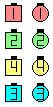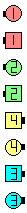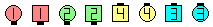Rotate 1/4\$B\$NA0(B As Couples 1/4 To Promenade\$B\$N8e(B Counter Rotate 1/4\$B\$N8e(B (\$B=*\$o\$j(B)
Single Rotate 1/4 (
from Columns EN: 480
Column \$B\$+\$i(B JP: 480
):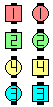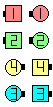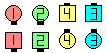Single Rotate 1/4\$B\$NA0(B 1/4 To Promenade\$B\$N8e(B Counter Rotate 1/4\$B\$N8e(B (\$B=*\$o\$j(B)

Single Rotate 1/4 (

from a T-Bone 2 x 4 EN: 500
T-Bone \$B\$N(B 2 x 4 \$B\$+\$i(B JP: 500
):

In this example, the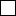dancers work as if they are in Columns whereas the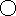dancers work as if they are in Lines (i.e., work Split) EN: 510
\$B\$3\$NNc\$G\$O(B,\$B\$N?M\$O(B Column \$B\$K\$\$\$k\$H\$7\$FF0\$-(B,\$B\$N?M\$O(B Line \$B\$K\$\$\$k\$H\$7\$FF0\$-(B (\$B\$9\$J\$o\$A(B, Split \$B\$G(B) \$B\$^\$9(B. JP: 510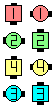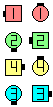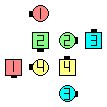before Single Rotate 1/4 after Some work Split: EN: 520 Some work Split: JP: 520 all 1/4 To Promenade Some work Split:all EN: 530 Some work Split:all JP: 530 Counter Rotate 1/4 (\$B=*\$o\$j(B)

Split Single Rotate 1/4 (

from Columns EN: 540
Column \$B\$+\$i(B JP: 540
):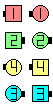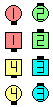before Split Single Rotate 1/4 after Split 1/4 To Promenade after Split Counter Rotate 1/4 (\$B=*\$o\$j(B)

Note: from Parallel Lines, Split Single Rotate is the same as Single RotateEN: 550
\$BCmSplit Single Rotate \$B\$O(B Single Rotate \$B\$HF1\$8\$G\$9(B. JP: 550

\$B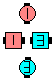\$B\$B;O\$a\$N(B formation: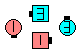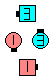after Single Rotate 1/4 after Diamond Single Rotate 1/4 ( See Diamond anything Concept [C4])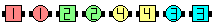\$B\$B;O\$a\$N(B formation: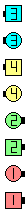Single Rotate 1/4\$B\$N8e(B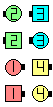Split Single Rotate 1/4\$B\$N8e(B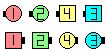Split Split Single Rotate 1/4\$B\$N8e(B

Hints for dancing Rotates: EN: 560
Rotates \$B\$rMY\$k\$?\$a\$N%R%s%H(B: JP: 560
• On Rotate or Single Rotate, turn 1/4 (As Couples or Individually) so that your Left-shoulder is toward the center of your setup. EN: 570
Rotate \$B\$^\$?\$O(B Single Rotate \$B\$G\$O(B, \$B:88*(B\$B\$r<+J,\$N(B setup \$B\$N(B center \$B\$NJ}\$XF0\$+\$9\$h\$&\$K(B 1/4 \$B\$=\$N>l\$G2s\$j\$^\$9(B (\$B%+%C%W%k\$+(B 1 \$B?M\$G(B). JP: 570
• On Reverse Rotate or Reverse Single Rotate, turn 1/4 (As Couples or Individually) so that your Right-shoulder is toward the center of your setup. ('Reverse' and 'Right' both begin with 'R'.) EN: 580
Reverse Rotate \$B\$^\$?\$O(B Reverse Single Rotate \$B\$G\$O(B, \$B1&8*(B\$B\$r<+J,\$N(B setup \$B\$N(B center \$B\$NJ}\$XF0\$+\$9\$h\$&\$K(B 1/4 \$B\$=\$N>l\$G2s\$j\$^\$9(B (\$B%+%C%W%k\$+(B 1 \$B?M\$G(B). ('Reverse' \$B\$H(B 'Right' \$B\$O(B, \$BN>J}(B 'R' \$B\$G;O\$^\$C\$F\$\$\$^\$9(B.) JP: 580
• Rotate means As Couples; Single Rotate means IndividuallyEN: 590
Rotate \$B\$O(B \$B!V%+%C%W%k\$G!W(B; Single Rotate \$B\$O(B \$B!V(B1 \$B?M\$G!W(B \$B\$r0UL#\$7\$^\$9(B. JP: 590
• If no fraction is given, then assume 1/4. In our opinion, the caller should always give a fractionEN: 600
\$B\$b\$7(B, fraction \$B\$,\$J\$\$\$H\$-\$O(B, 1/4 \$B\$H\$7\$F\$/\$@\$5\$\$(B. \$B;dC#\$N0U8+\$G\$O(B, \$B%3!<%i!<\$O>o\$K(Bfraction \$B\$rM?\$(\$k\$Y\$-\$G\$9(B. JP: 600
• The caller can give the fraction 0 (or 0/4), in which case just turn 1/4 and do not Counter Rotate at all. EN: 610
\$B%3!<%i!<\$O(B fraction 0 (\$B\$^\$?\$O(B 0/4) \$B\$H\$9\$k\$3\$H\$,\$G\$-\$^\$9(B. \$B\$3\$N>l9g(B, \$B\$=\$N>l\$G(B 1/4 \$B2s\$k\$@\$1\$G(B, Counter Rotate \$B\$O2?\$b\$7\$^\$;\$s(B. JP: 610

Why is the Rotate FAMILY split across three levels? The answer is that the definition of Rotate is inconsistent, in the sense that the definition is formation-dependent. In our opinion, dancers should have one concise definition that can be applied to any legal starting formation. The C2-style Rotates are the culprits, and we believe these Rotates should have been named Split Rotate (instead of Rotate), and Split Single Rotate (instead of Single Rotate), so that there would be no conflict. Unfortunately, Rotates were not defined quite so cleanly. EN: 620
\$B\$J\$<(B, Rotate FAMILY \$B\$O(B 3 \$B\$D\$N%l%Y%k\$K\$^\$?\$,\$C\$F\$\$\$k\$N\$G\$7\$g\$&\$+(B? \$BEz\$(\$O(B, Rotate \$B\$NDj5A\$,(B formation \$B\$K0MB8\$7\$F\$\$\$F(B, \$B0l4S@-\$,\$J\$\$\$+\$i\$G\$9(B. \$B;dC#\$N0U8+\$G\$O(B, \$BDj5A\$O%@%s%5!<\$K\$H\$C\$F(B 1 \$B\$D\$N4J7i\$J\$b\$N\$G(B, \$B\$I\$NE,@Z\$J;O\$a\$N(B formation \$B\$+\$i;H\$(\$k\$b\$N\$G\$"\$k\$Y\$-\$G\$9(B. C2 \$B%9%?%\$%k\$N(B Rotates \$B\$K\$OLdBj\$,\$"\$j(B, \$B\$3\$l\$i\$N(B Rotates \$B\$O(B Split Rotate (Rotate \$B\$G\$J\$/(B) \$B\$H(B Split Single Rotate (Single Rotate \$B\$G\$J\$/(B) \$B\$HL>\$E\$1\$k\$Y\$-\$G\$7\$?(B, \$B\$=\$&\$9\$l\$PL7=b\$,\$"\$j\$^\$;\$s(B. \$B;DG0\$J\$,\$i(B, Rotates \$B\$O\$-\$A\$s\$H@0M}\$7\$FDj5A\$5\$l\$^\$;\$s\$G\$7\$?(B. update JP: 620

\$BCounter Rotate [C1].CALLERLAB definition for RotateCALLERLAB definition for Reverse RotateCALLERLAB definition for Single RotateCALLERLAB definition for Reverse Single RotateComments? Questions? Suggestions?

 C1: C2: C3B:https://www.ceder.net/def/rotate_c1.php?level=C1,C2,C3B&language=japan&action=edit
06-December-2021 13:08:42